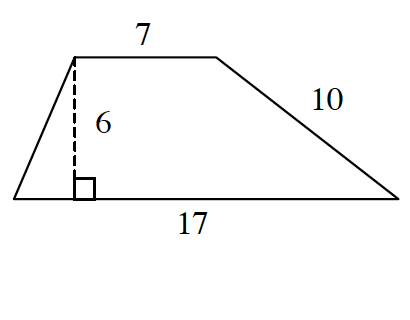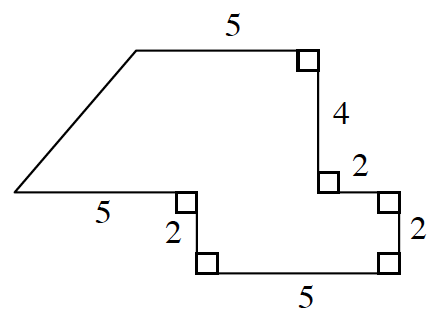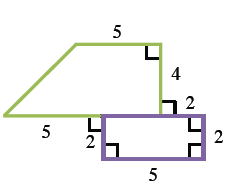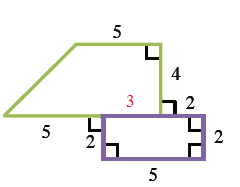### Home > GC > Chapter 5 > Lesson 5.3.5 > Problem5-117

5-117.

Find the area and perimeter of each shape below. Show all work.

1.2.Divide the figure into two separate shapes.
Afterwards, find the area for each shape and then add them together.

The piece shared between the trapezoid and the rectangle has a length of $5-2=3$ units because the purple rectangle has a side length of $5$ units. This makes the base of the trapezoid $8$ units.

Area of Shape = Area of Trapezoid + Area of Rectangle

$\frac{1}{2}\left(\textit{b}_1+\textit{b}_2\right)+\textit{bh}$

$\frac{1}{2}([5+3]+5)4+2\cdot 5$

$\text{Total Area}=36\text{ units}^2$